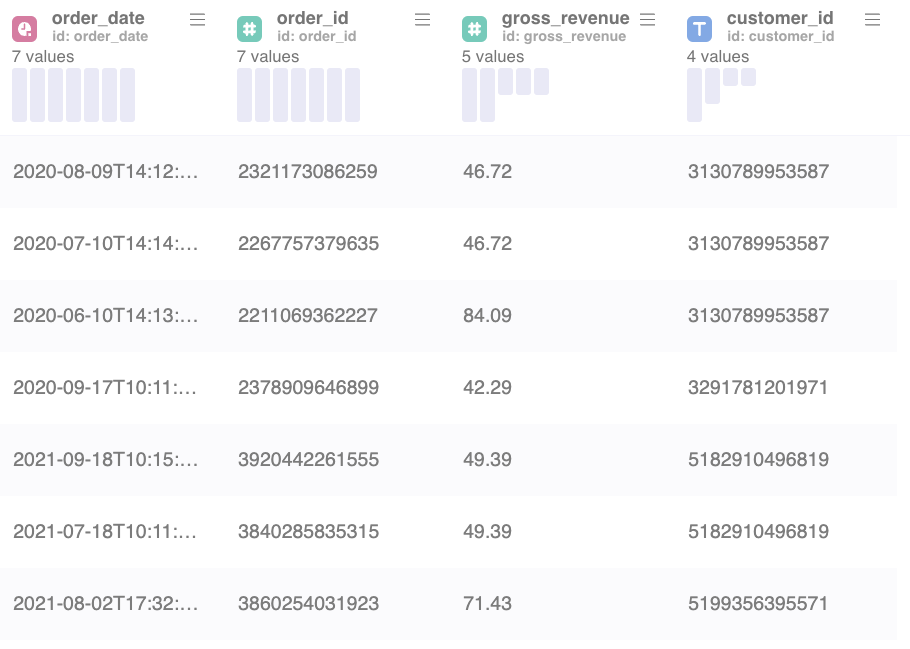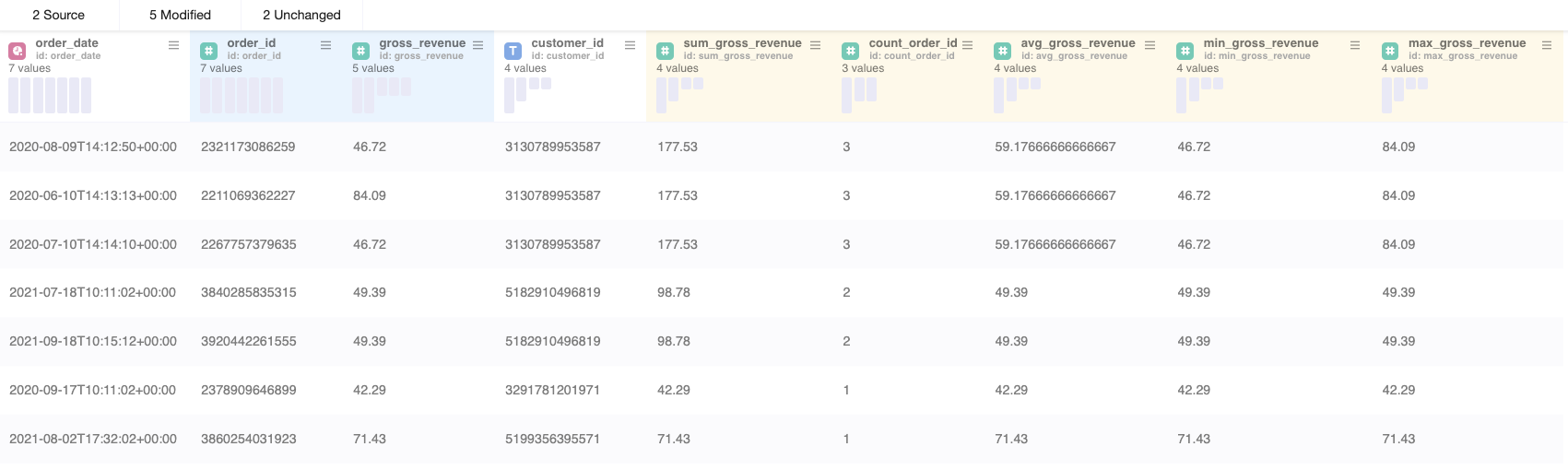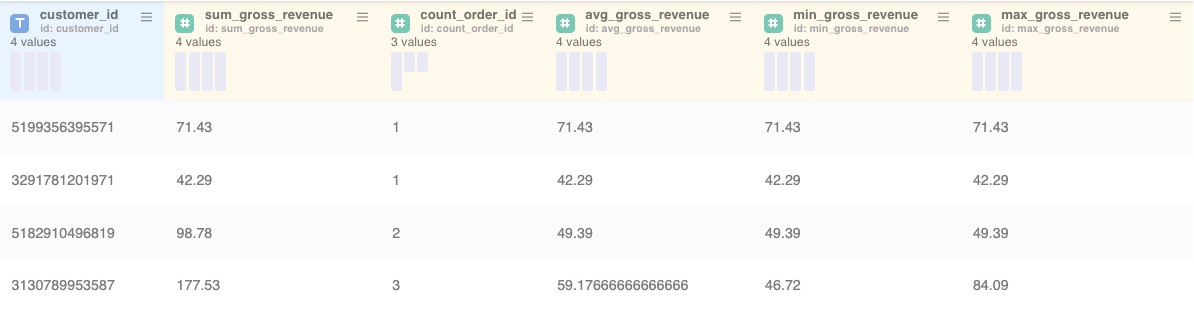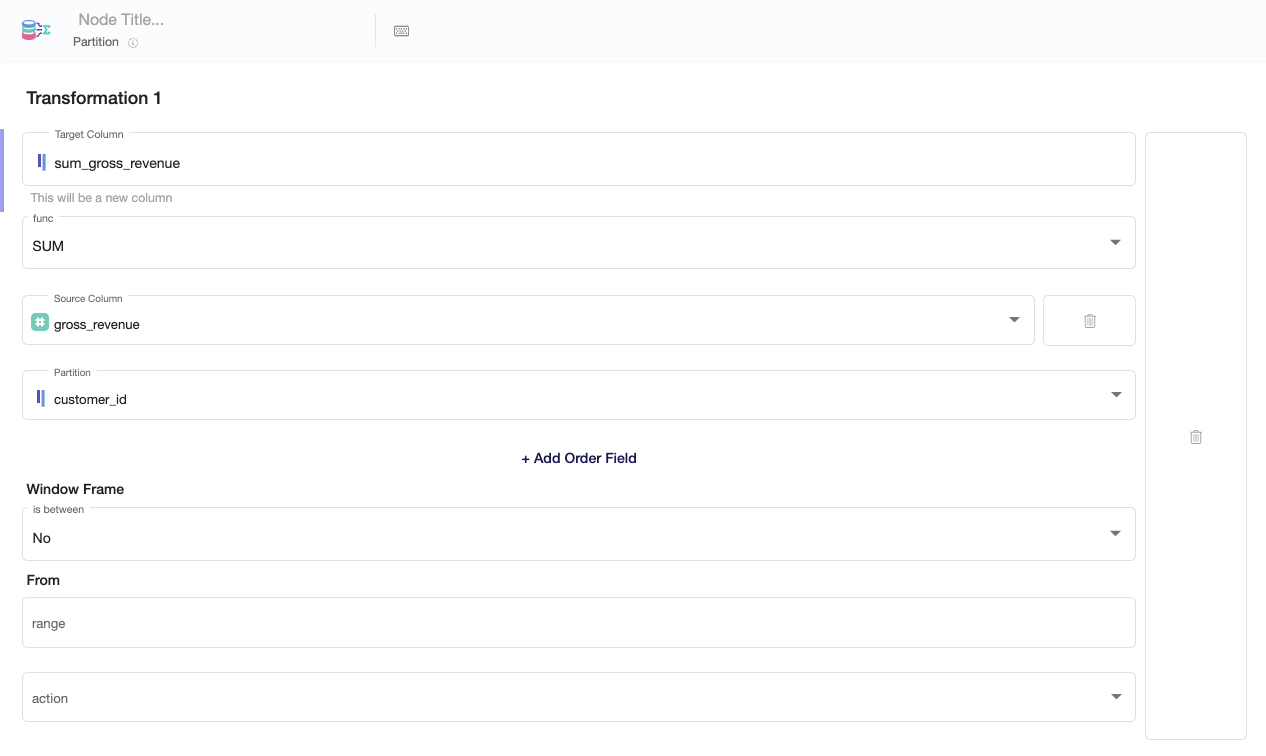The Partition node allows you to perform calculations over a set of rows which are related to each other. Such a calculation can be for example: SUM of revenue by customer.

Other than the Aggregation node, the Partition node does not collapse original rows but keeps the structure of the table as before.

### Understanding the Partition node

The Partition node can group values by certain criteria similar to the Aggregation node. The possible grouping functions are

• SUM
• COUNT
• AVG
• MIN
• MAX

Example 1:Example 1 is showing a table with transactions including transaction details such as gross_revenue, order_date and customer_id. Every row is a transaction done by a customer.

When using the PARTITION node to calculate following values for each customer_id

• SUM of gross_revenue
• COUNT of order_id's
• AVG of gross_revenue
• MIN of gross_revenue
• MAX of gross_revenue

The result would look as follow:

Example 2:With the Partition node the structure of the table remains and the values which got aggregated by customer_id were added as new columns.

The same aggregation with the Aggregation node would have output the following result:

Example 3:Example 3 shows the aggregation by customer_id with the usage of the Aggregation node. The resulting table is a collapsed table where gross_revenue and count of order_id's got aggregated on customer_id level.

### Window functions

Besides of the aggregation functions shown above, the Partition node also offers more powerful window functionalities such as

• ROW_NUMBER - This function numbers the rows according to a custom-defined order. In combination with a "partition by" definition you can begin counting at 1 again in each partition.
• RANK - This function is similar to the ROW_NUMBER function. The difference is that RANK gives the same rank in case of same order value's. When multiple rows share the same rank, the rank of the next row is not consecutive. This is similar to Olympic medaling in that if two athletes share the gold medal, there is no silver medal.
• DENSE_RANK - This function assigns ranks to rows in partitions with no gaps in the ranking values (other than the RANK function). If two or more rows in each partition have the same values, they receive the same rank. The next row has the rank increased by one. Different from the RANK function, the DENSE_RANK function always generates consecutive rank values.
• PERCENT_RANK - This function calculates the percentile ranking of rows in a result set. It returns a percentile ranking number which ranges from zero to one. For the first row it always returns zero.
• CUM_DIST - This function calculates the cumulative distribution of a value within a window or partition.
• NTILE - This function identifies what percentile (or quartile, or any other subdivision) a given row falls into

### Setting up your Partition node1. Select the Target Column you wish to modify or write the name of a new column.
2. Choose the functionality of the Partition node you want to use (SUM, COUNT, AVG ...)
3. Choose the Source Column on which you will perform the choosen function
4. Choose the column you want to partition by (the table will be divided into groups/partitions and and the function will be performed on each subset of the partitioned data)

In the following video you can see an example use of the Partition node with usage of the RANK function:

https://www.loom.com/embed/cea32289dff34f609e40df3fb4c4eb99

### Window frame

An additional option is to create a window frame within a partition. Window frames are a feature that allows us to divide partitions into smaller subsets. What’s even more important, these subsets can differ from row to row. This is something that can’t be achieved with partitioning only.

In the following video you can see an example use case of the Partition node with usage of window frames and the AVG function:

https://www.loom.com/embed/1d43a94343d24ffbb6ddd73b4309bc44